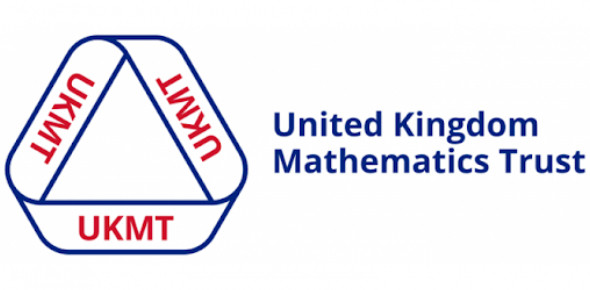UK Mathematics Trust (UKMT) Maths Challenge Quiz

10 Questions | Total Attempts: 87SettingsMaths isn't just multiplication and addition, it's also about thinking logically. UKMT Maths designs to make you think deeply and not just make you guess answers. So, if you want to compete in this challenge, test yourself first with this quiz.

• 1.
This 3 by 3 grid shows nine 1 cm × 1 cm squares and uses 24 cm of wire. What length of wire is required for a similar 20 by 20 grid?
• A.

400

• B.

420

• C.

441

• D.

800

• E.

840

• 2.
Four different positive integers have a product of 110. What is the sum of the four integers?
• A.

19

• B.

22

• C.

24

• D.

25

• E.

28

• 3.
Look at the grid on the right. Molly starts at the star. She moves 2 squares south-west, 2 squares north and one square east. What shape will she arrive at?
• 4.
Two players X and Y take alternate turns in a game, starting with the diagram alongside. At each turn, one player writes one of 1, 2 or 3 in an empty circle, so that no two circles connected by an edge contain the same number. A player loses when they cannot go. In each of the five diagrams below, it is Y’s turn. In which of the diagrams, can Y’s move ensure that X loses the game?
• A.

Diagram 1

• B.

Diagram 2

• C.

Diagram 3

• D.

Diagram 4

• E.

Diagram 5

• 5.
In the figure shown, each line joining two numbers is to be labelled with the sum of the two numbers that are at its end points. How many of these labels are multiples of 3?
• A.

10

• B.

9

• C.

8

• D.

7

• E.

6

• 6.
How many of the four Knaves were telling the truth?
• 7.
In the window of Bradley's Bicycle Bazaar, there are some unicycles, some bicycles and some tricycles. Laura sees that there are seven saddles in total, thirteen wheels in total and more bicycles than tricycles. How many unicycles are in the window?
• A.

1

• B.

2

• C.

3

• D.

4

• E.

5

• 8.
I add up all even numbers between 1 and 101. Then from my total, I subtract all odd numbers between 0 and 100. What is the result?
• A.

0

• B.

50

• C.

100

• D.

255

• E.

2525

• 9.
There are ——— vowels in this short sentence. Which of the following options should replace "———" to make the sentence in the box true?
• A.

Twelve

• B.

Thirteen

• C.

Fourteen

• D.

Fifteen

• E.

Sixteen

• 10.
Ali wants to fill the empty squares, so that the number in each square after the fourth from the left is the sum of the numbers in the four squares to its left. What number should Ali write in the final square?
• A.

16

• B.

8

• C.

4

• D.

2

• E.

1

Related TopicsBack to top